﻿﻿ Coil-to-Coil Power Transferexamples｜products｜Murata Software Co., Ltd.# Example33Coil-to-Coil Power Transfer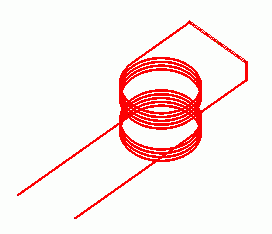### General

• The power is transfered from the primary coil to the secondary coil.

• The magnetic field vectors and the electromotive force in the secondary coil are solved.
If you want to solve the transfer power [W], use the electromagnetic waves analysis [Hertz]. ([Exercise 28: Coil-to-Coil Power Transfer])

• Unless specified in the list below, the default conditions will be applied.

### Analysis Space

 Item Settings Analysis Space 3D Model unit mm

### Analysis Conditions

 Item Setting Solver Magnetic Field Analysis [Gauss] Analysis Type Harmonic analysis Options N/A

The frequency of the current is set to 30[kHz].

 Tab Item Setting Mesh Tab Frequency-Dependent Meshing Reference frequency: 30×10^3[Hz] Harmonic analysis Sweep Type Single frequency Frequency 30×10^3[Hz]

Set the Mesh Tab as follows.

 Tab Item Setting Mesh Tab Meshing Setup Automatically set the general mesh size: Deselect General mesh size: 2[mm]

### Model

Two coils (Coil1 and Coil2) are placed.

Its inflow/outflow faces if the primary coil (Coil1) are extended to the outside of the ambient air. They contact the electric wall of outer boundary condition.

In the magnetic field harmonic analysis, inflow/outflow faces must be outside of the ambient air. (for the purpose of calculation with FEM)

In this Exercise, ambient air is set to be created automatically. Therefore, the inflow/outflow faces are extended to the outside of ambient air.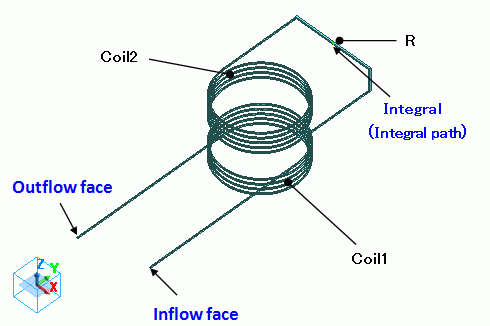Secondary coil (Coil2) is terminated with R having sufficient resistance value to measure the electromotive force.

If the boundary condition of integral path is set along the current path, the electromotive force can be calculated by integrating the electric field (current density*resistance) on the integral path.

Basically, the body R is not needed when setting the integral path along the current path of the loop coil. However, the current density is not constant at the section of the coil and calculation cannot be done accurately.

By adding the body R having resistance high enough compared to the coil,

the electric potential difference is generated mostly at the body R, and the current density at the section of the body R is almost constant. So the calculation will be accurate.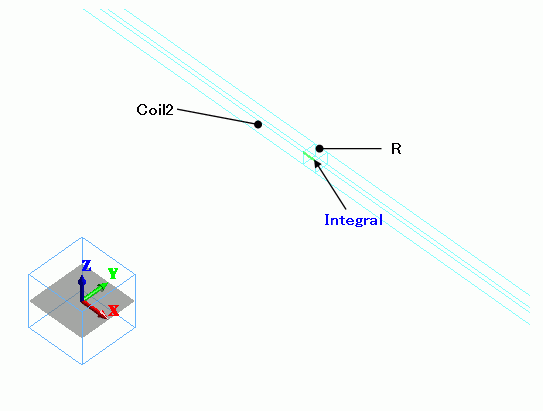Body Number/Type Body Attribute Name Material Name 4/Solid Coil1 008_Cu * 11/Solid Coil2 008_Cu * 9/Solid R 008_Cu *

* Available from the Material DB

Body attribute of the primary coil is set up as follows to apply the current.

The setting of the secondary coil is not needed as the current is not applied.

 Body Attribute Name Tab Setting Coil1 Current Waveform: AC Current: 1[A] Turns: 1[Turns] Direction: Specify Inflow/Outflow Faces Select inflow face and outflow face.

The electric conductivity of the body R is set low enough compared to the coil as follows.

 Material Name Tab Setting R Conductor wall Conductivity Type: Conductor Conductivity: 1[S/m]

No setting.

### Results

To see the electromotive force, go to the [Results] tabclick [Table]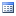.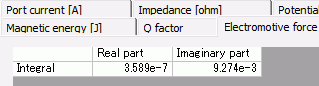The absolute value is the amplitude of the electromotive force.

About 0.00927[V] is generated in the secondary winding when 1[A] is applied in the primary winding.

The electromotive Ve.m. of the secondary coil is expressed as follows.

Ve.m. = jωM I1

As the frequency is 30kHz, the mutual inductance is 0.491nH (the results of [Mutual inductance calculation project]), and the current I1 at Coil1 is 1A,
amplitude |Ve.m.| of the electromotive force of the secondary coil is calculated to be 0.00925V. This matches with the output result.

• If you want to solve the transfer power [W], use the electromagnetic waves analysis [Hertz]. ([Exercise 28: Coil-to-Coil Power Transfer])

The vectors of the magnetic field are shown below.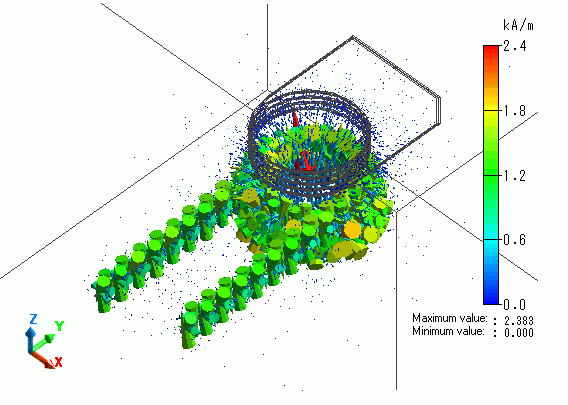﻿ ﻿